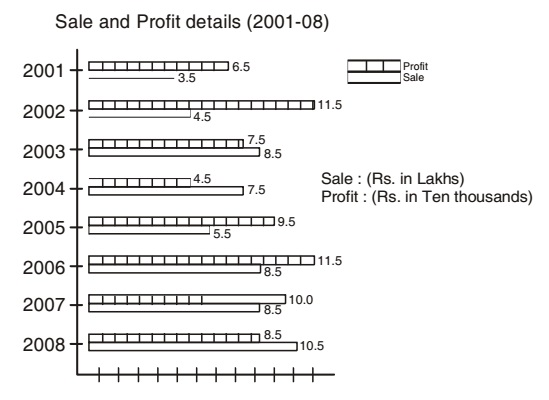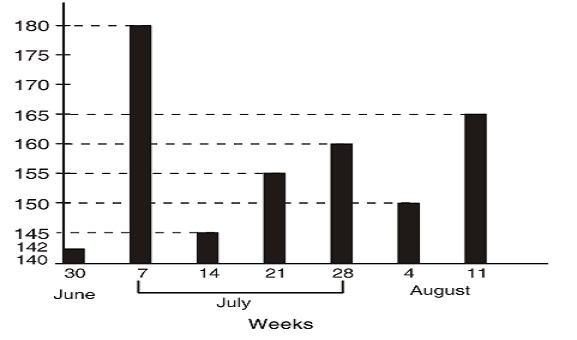## Bar graph

#### Data Interpretation

Direction: The bar graph as shows below gives information about the sale and profit details of a departmental store during the years from 2001 - 2008. Study the graph carefully and answer the questions asked here under.1. Annual mean profit of the store (rupees in ten thousand) is approximately:

1. Annual mean profit of the store (rupees in ten thousands)
= Add the annual income of every years / difference of the year

##### Correct Option: C

Annual mean profit of the store (rupees in ten thousands)
= (6.5 + 11.5 + 7.5 + 4.5 + 9.5 + 11.5 + 10.0 + 8.5)/8 = 69.5/8 =8.7 (approx.)

1. During which of the following years percentage of profit earned by the store on the total sale was at the highest level?

1. Percentage of profit on the total in 2001 = Total profit in rupees x 100/ total sale in rupees.

##### Correct Option: B

Percentage of profit on the total in 2001 = Total profit in rupees x 100/ total sale in rupees.
Percentage of profit on the total in 2001 = 6.5 x 10000 x 100/3.5 x 100000 = 18.57
Percentage of profit on the total in 2002 = 11.5 x 10000 x 100/4.5 x 100000 = 25.56
Percentage of profit on the total in 2003 = 7.5 x 10000 x 100/8.5 x 100000 = 8.82
And Percentage of profit on the total in 2005 = 9.5 x 10000 x 100/ 8.5 x 100000 = 8.82
∴ Percentage of profit on the total sale in 2002 was the highest level.

1. Assuming the profit earned during the year 2001 as base (100), the profit made by the store during the year 2008 was:

1. Required profit = 100 x 85 /6.5 = 131

##### Correct Option: D

Required profit = 100 x 85 /6.5 = 131

1. During which year between 2001 to 2006 profit made by the store as compared to the previous year was more than 100%?

1. Profit per cent during the year 2005 as compared to the previous year =(95 - 45) x 100/4.5

##### Correct Option: C

Profit per cent during the year 2005 as compared to the previous year =(95 - 45) x 100/4.5 = 111.11%
Hence, profit in the year 2005 was more than 100% as compared to the previous year.

Direction: The bar graph given here represents the number of persons killed in road accidents during rainy season in the year 2009. Study the graph carefully and answer the questions that follow:1. During which of the following successive weeks number of persons killed was the highest?

1. According to the graph, the two successive weeks vis., 30 June - 7 July shows highest increase.

##### Correct Option: C

According to the graph, the two successive weeks vis., 30 June - 7 July shows highest increase of 180 - 142 = 38 in the number of persons killed.